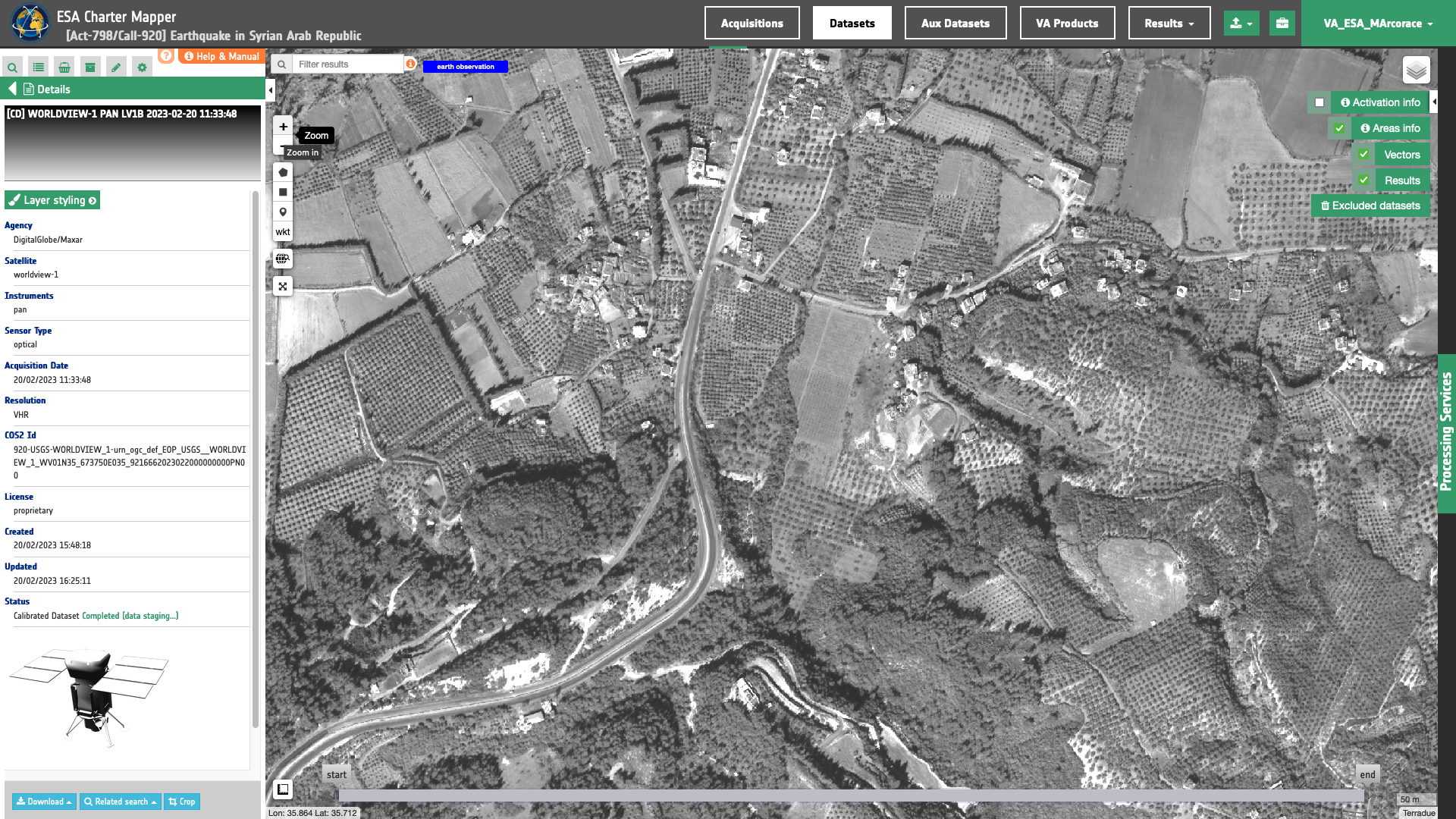# WorldView-1

WorldView-1 L1B panchromatic EO data products are calibrated using the Optical Products Calibration (OPT-Calib) service.## Input

The input is an ingested WorldView-1, find details here.

The WorldView-1 product handbook1 provides the formula below to convert the DN into radiance:

$L_{(b)} = gain_{(b)} \times DN_{(b)} \times { abscalfactor \over effectivebandwith } + offset_{(b)}$

Where:

$$gain_{(b)}$$ and $$offset_{(b)}$$ are values taken from the table below:

Band Gain Offset
pan 1.016 -1.824

Table 1 - Gain and offset for the Worldview-1 DN to radiance conversion.

And $$abscalfactor$$ and $$effectivebandwith$$ are extracted from the acquisition metadata.

The conversion from $$DN$$ (for Digital Numbers) to spectral radiance (or ‘TOA radiance’) $$L$$ is done with the formula below:

$L_\lambda = gain \times DN + offset$

As such, the calibration uses:

$gain = gain_{(b)} \times { abscalfactor \over effectivebandwith }$

and

$offset = offset_{(b)}$

$$L$$ in $$W/m^2/steradians/micrometers$$ with $$b$$ being a band ID.

To convert TOA radiance to TOA reflectance, the following formula is used :

$R(b) = { {\pi \times L(b) \times d \times d} \over {ESUN(b) \times cos(θ)} }$

where :

• $$L(b)$$ is the spectral radiance for band b

• $$pi$$ is the famous mathematical constant

• $$d$$ is the earth-sun distance (in astronomical units) and depends on the acquisition’s day and month

• $$ESUN(b)$$ is the mean TOA solar irradiance (or solar illumination) in $$W/m^2/micrometers$$

• $$θ$$ is the solar zenith angle in degrees.

The conversion from elevation angle to solar zenith angle $$θ$$ (in degrees) is:

$θ = { 90 - elevation_angle }$

The value for the $$ESUN(b)$$ is provided in Table 2:

Common band name Thuillier 2003
pan 1478.62

Table 2 - ESUN value employed in the conversion of radiance to reflectance for Worldview-1 products.

## Output

The output is a STAC item GeoJSON2 having a similar structure to the Worldview-3 one available here.

1. Kuester, M. Absolute Radiometric Calibration: 2016v0; updated on 6/6/2017 GE01 and CAVIS, DigitalGlobe: Westminster, CO, USA, 2017; pp. 1–8.

2. Radiant Earth Foundation, STAC item specifications, a single spatiotemporal asset as a GeoJSON feature plus datetime and links, available at:https://github.com